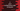# C program to print multiplication table using goto statement## C program to print multiplication table using goto statement:

In this post, we will use goto statement of C programming language to print the multiplication table for a user. This program will ask the user to enter a number, it will read that number and print one multiplication table using goto for that number.

### How goto works in C:

goto is used to move the control to a different point in the same function using a label. The control moves to the label pointed by goto and code execution starts from that label.

For example, let’s consider the below program:

``````#include <stdio.h>

int main()
{
printf("First line\n");
goto third_line;

printf("Second line\n");

third_line:
printf("Third line\n");
}``````

Here, we have three printf statements. But we are using goto after the first printf statement. That will move the control to third_line and execute the third printf statement without running second printf.

If you run this program, it will print the below output:

``````First line
Third line``````

### Printing the multiplication table using goto:

Let’s try to print the multiplication table using goto. We will take one number from the user and print the multiplication table for that number using goto :

``````#include <stdio.h>

int main()
{
int no;

printf("Enter a number : ");
scanf("%d", &no);

int currentLine = 1;

table:
printf("%d * %d = %d\n", no, currentLine, no * currentLine);
currentLine++;

if (currentLine < 11)
{
goto table;
}
}``````

Here,

• no variable is used to hold the number entered by the user.
• currentLine is used to note down the current line number. It is 1 in the beginning. We will run till it is 10.
• table is the start point of printing the multiplication table. We are printing one line of the table, incrementing the value of currentLine by 1 and if its value is less than 11, moving back to table using goto. So, it loops in the table part to print down each line of the multiplication table.

### Sample output:

It will print output as like below:

``````Enter a number : 5
5 * 1 = 5
5 * 2 = 10
5 * 3 = 15
5 * 4 = 20
5 * 5 = 25
5 * 6 = 30
5 * 7 = 35
5 * 8 = 40
5 * 9 = 45
5 * 10 = 50

Enter a number : 10
10 * 1 = 10
10 * 2 = 20
10 * 3 = 30
10 * 4 = 40
10 * 5 = 50
10 * 6 = 60
10 * 7 = 70
10 * 8 = 80
10 * 9 = 90
10 * 10 = 100``````